##### Actions

(diff) ← Older revision | Latest revision (diff) | Newer revision → (diff)

A theorem providing an upper bound of the value of a Vinogradov integral: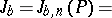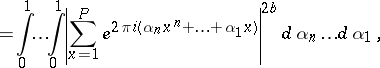whereis the average value of the trigonometric sum. It is formulated as follows. If, for a non-negative integerone sets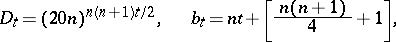then, ifand for an integer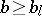,The estimate of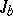given by Vinogradov's theorem is asymptotically exact. The theorem is fundamental in the Vinogradov method for estimating Weyl sums (cf. Weyl sum). In addition, it yielded a number of almost optimal results in classical problems in number theory (cf. Waring problem; Hilbert–Kamke problem; Distribution modulo one of a polynomial).

How to Cite This Entry: